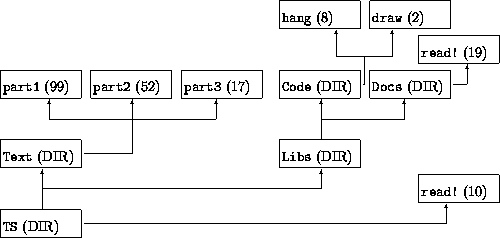#### Filesystem

This assignment is borrowed directly from How to Design Programs. Please note that it would be a violation of the Academic Code to look at the corresponding material in that book and use any content associated with it there (not that it will necessarily help you).

##### 2Background

The goal of this assignment is to model a filesystem. A filesystem is that part of the computer that remembers programs and data when the computer is turned off (i.e., it makes the data persistent).

On most computer systems, the collection of files is organized in directories. Roughly speaking, a directory contains some files and some more directories. The latter are called subdirectories and may contain yet more subdirectories and files, and so on. The entire collection is collectively called the filesystem.This figure shows a small filesystem. Each box has one of two annotations. A directory is annotated with DIR, and a file is annotated with a number, which signifies the file’s size. The tree’s root directory is TS. It contains one file, read!, and two subdirectories, Text and Libs. The Text subdirectory contains three files; Libs contains two subdirectories, each of which contains at least one file. Altogether, TS contains seven files and consists of five (sub)directories.

##### 3Data Representation

Now let’s model a filesystem in Pyret. Since a directory contains files and directories, we will need data structures to represent both of those. First, we define a data structure for files:
 data File: | file(name :: String, size :: Number, content :: String) end
Every file has a name, some content, and a size based on the content.On a real computer, all the parts of a filesystem can change dynamically. For simplicity, we will ignore this aspect here. Assume that the size field is automatically kept up-to-date with the file’s content.

A directory then has a name, a list of files, and a list of (sub)directories:
 data Dir: | dir(name :: String, ds :: List, fs :: List) end
We already know how to model Lists, so we elide the definition of these lists here.

This models a typical filesystem. Based on this model we can create functions that approximate the behavior of an actual computer.

##### 4Exercises
1. Develop the function

how-many :: Dir -> Number

which consumes a Dir and produces the number of files in the directory tree.

2. Develop the function

du-dir :: Dir -> Number

The function consumes a directory and computes the total size of all the files and subdirectories. Assume that storing a file and a directory in a dir structure costs 1 storage unit. The size of a directory is the sum of the sizes of its contents, the length of its files list, and the length of its dirs list. For example, in the figure, the size of the TS directory is 218, and the size of the Code is 12.

3. Develop the function

can-find :: Dir, String -> Boolean

which consumes a Dir and a file name (String) and determines whether or not a file with this name occurs anywhere in that directory tree.

4. Develop the function

fynd :: Dir, String -> List<Path>

(so-named because Pyret has a function called find built in). It consumes a Dir d and a file name (String) f, and produces a list of all the paths to all the files named f in d. (That means, if can-find(d, f) is false, it produces empty.)

A path is a list of directory names. The first one is that of the given directory; the last one is that of the subdirectory that contains f. Each path should lead to a different occurrence, and there should be a path for each occurrence. To make this easier for you, we have defined the data type Path to be a list of strings (directory names), so fynd should return List<Path>.

For example, in the figure:

 check: fynd(TS, "part3") is [list: [list: "TS", "Text"]] fynd(TS, "read!") is [list: [list: "TS"], [list: "TS", "Libs", "Docs"]] end

Examplar

Program

Final Tests

##### 8Handing In

Filenames: filesystem-code.arr, filesystem-tests.arr

Submission